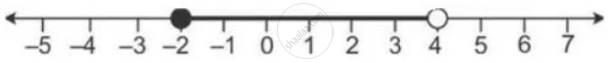Share

Solve the Given Inequation and Graph the Solution on the Number Line. 2y – 3 < Y + 1 ≤ 4y + 7, Y ∈ R - ICSE Class 10 - Mathematics

ConceptSolving Algebraically and Writing the Solution in Set Notation Form

Question

Solve the given inequation and graph the solution on the number line.
2y – 3 < y + 1 ≤ 4y + 7, y ∈ R

Solution

2y – 3 < y + 1 ≤ 4y + 7, y ∈ R

⇒ 2y – 3 – y < y + 1 – y ≤ 4y + 7 – y

⇒ y – 3 < 1 ≤ 3y + 7

⇒ y – 3 < 1 and 1 ≤ 3y + 7

⇒ y < 4 and 3y ≥ 6 ⇒ y ≥ – 2

⇒ – 2 ≤ y < 4

The graph of the given equation can be represented on a number line as:Is there an error in this question or solution?

APPEARS IN

Solution Solve the Given Inequation and Graph the Solution on the Number Line. 2y – 3 < Y + 1 ≤ 4y + 7, Y ∈ R Concept: Solving Algebraically and Writing the Solution in Set Notation Form.
S